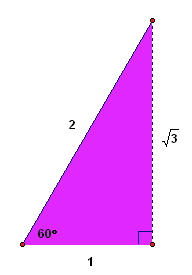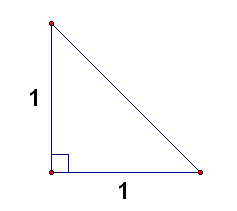﻿ Two Special Triangles

#Two Special Triangles

You should know the relationship between the lengths of the sides in two special triangles:

• $\,30^\circ{-}60^\circ{-}90^\circ\,$ triangles
• $\,45^\circ{-}45^\circ{-}90^\circ\,$ triangles

Lengths of Sides in a $\,30^\circ\text{-}60^\circ\text{-}90^\circ\,$ Triangle
Let $\,s\,$ denote the length of the shortest side. Then, the hypotenuse has length $\,2s\,.$ The side opposite the $\,60^\circ\,$ angle has length $\,\sqrt{3}s\,.$

Conversely, if a triangle has sides of lengths $\,s\,,$ $\,\sqrt{3}s\,,$ and $\,2s\,,$ then it is a $\,30^\circ\text{-}60^\circ\text{-}90^\circ\,$ triangle.

Since $\,\sqrt{3}\approx 1.7\,,$ it follows that the side opposite the $\,60^\circ\,$ angle is a little more than one and a half times the shortest side.

Lengths of Sides in a $\,45^\circ\text{-}45^\circ\text{-}90^\circ\,$ Triangle
Let $\,s\,$ denote the length of the two shorter sides. Then, the hypotenuse has length $\,\sqrt{2}s\,.$

Conversely, if a triangle has sides of lengths $\,s\,,$ $\,s\,,$ and $\,\sqrt{2}s\,,$ then it is a $\,45^\circ\text{-}45^\circ\text{-}90^\circ\,$ triangle.

Since $\,\sqrt{2}\approx 1.4\,,$ it follows that the hypotenuse is a little less than one and a half times the shortest side.

It's easy to see that these are the correct relationships between the lengths of the sides, as follows:

## $\,30^\circ\text{-}60^\circ\text{-}90^\circ\,$ Triangle Relationships

Start with an equiangular (hence equilateral) triangle, where each side has length $\,2\,.$ Notice that all the angles must be $\,\frac{180^\circ}{3} = 60^\circ\,.$

Drop a perpendicular, as shown below. The purple and green triangles are $\,30^\circ\text{-}60^\circ\text{-}90^\circ\,$ triangles, where the length of the shortest side is $\,1\,.$A quick application of the Pythagorean Theorem shows that the remaining side has length $\,\sqrt{3}\,.$ Then, scale all sides by $\,s\,.$

## $\,45^\circ\text{-}45^\circ\text{-}90^\circ\,$ Triangle Relationships

Create a triangle with two sides of length $\,1\,$ and a $\,90^\circ\,$ angle, as shown below.Since angles opposite equal sides must be equal, and since the two remaining angles must add to $\,90^\circ\,,$ they must each be $\,45^\circ\,.$

A quick application of the Pythagorean Theorem shows that the remaining side must equal $\,\sqrt{2}\,.$ Then, scale all sides by $\,s\,.$

## Examples

Question: In a $\,30^{\circ}\text{-}60^{\circ}\text{-}90^{\circ}\,$ triangle, suppose that the length of the hypotenuse is $\,70\,.$ What is the length of the side opposite the $\,60^{\circ}\,$ angle?
Solution: One strategy is to get the shortest side first, which is half the hypotenuse: the shortest side is $\,\frac{70}2 = 35\,.$ Then, the side opposite the $\,60^{\circ}\,$ angle is $\,\sqrt{3}\,$ times as long, hence is $\,35\sqrt 3\,.$
Question: Suppose that the lengths of the sides in a triangle are $\,13\,,$ $\,13\sqrt{2}\,,$ and $\,13\,.$ What type of triangle is this?
Solution: This is a recognition question. Notice that two sides are the same, and the other is $\,\sqrt{2}\,$ times as large. This is a $\,45^{\circ}\text{-}45^{\circ}\text{-}90^{\circ}\,$ triangle.